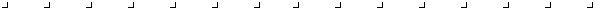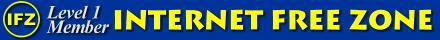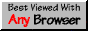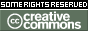Updated: August 11, 2004

# Catching Integer Overflow

If you do artithmetic with integers fed to your program from external sources, chances are you'll experience integer overflow. No run-time exception is normally generated for integer overflow, so you have to catch it yourself.

Here are some comments on how to do that.Submitted by: Paul G. Cumming di316@freenet.carleton.ca

```Here is the algorithm Motorola uses to correctly set the overflow bit

A1 = most significant bit (sign bit) of first addend
+ A2 = most signficiant bit of second addend
-----
S = most signficiant bit of sum

V = (A1 or A2 or (not S)) and ((not A1) or (not A2) or S)

This can be translated into C like this, with Operand1 and Operand2
being two integer or long addends, and Result being the sum: (Overflow is
the same data type and Operand1, Operand2, and Result:

Overflow = (Operand1 & Operand2 & ~Result) | (~Operand1 & ~Operand2 & Result);

If Overflow is less than 0, an overflow occurred during addition.

Checking for overflow during subtraction works like this (here overflow
means the _magnitude_ of the number is too great, so overflow (or
underflow) can occur if the result is to small to represented):

M - most significant bit (sign bit) of minuend
- S - most significant bit of subtrahend
----
D - most significant bit of difference

V = (M and (not S) and (not D)) or ((not M) and S and D)

Put in C, with Operand1 as the minuend, Operand2 as the subtrahend, and
Result as the difference:

Overflow = (Operand1 & ~Operand2 & ~Result) | (~Operand1 & Operand2 &
Result);

If Overflow is less than 0, an overflow occurred during subtraction.

I got these algorithms from a sheet handed out to me in a Computer
Architecture lab back in semester 3, called "Condition Code Computations."
You can probably find this information in some of the many books the
library has on Motorola's 680x0 family.  The algorithms for multiplication
and division overflow are not given.  I guess Motorola's doesn't want to
give away all of their trade secrets.  ;^)
Division overflow really doesn't exist with integer division.  The only
way to make a number larger when dividing is to divide it by a fraction,
which you can't do with integer division.
Multiplication overflow seems to be really hard to catch, but here's
something I thought up.  If the overflow doesn't go very far (only one
sign change), you can check for overflow by verifying the sign of the
result.
We already know, from grade school, what the sign of the result when
multiplying two numbers is:

Sign Truth Table
Factor 1    Factor 2   Product
+           +          +
-           +          -
+           -          -
-           -          +

Well, that looks an awful lot like the truth table for an exclusive OR
gate.

F1 = most significant bit (sign bit) of first factor
F2 = most significant bit of second factor
P  = most significant bit of product

V = ((F1 xor F2) != P)

This can also be done in C, but this time, you must use the sign bit,
not the whole number (because you need to make sure two numbers are of the
same sign -- in BASIC, you could use the SGN(x) function.  I don't think
there's an equivalent (for integers, at least) in C).

Overflow = (((Operand1 ^ Operand2) & LONG_MIN) != (Result & LONG_MIN));

LONG_MIN (from ANSI library <limits.h>) = lowest possible long integer
(which would be 1000 0000 0000 0000 0000 0000 0000 0000 in binary -- only
the sign bit is on).  Replace this with INT_MIN for integers.

If Overflow is 1, an overflow occurred.  If Overflow is 0, an overflow
_probably_ did not occur.```

## Commentary on the above

```From: Jonathan Gilbert logic at phayze dot com
Date: Sun, 08 Aug 2004 21:55:26 -0400
Subject: issues with integer overflow catching algorithms

Hello,

I did a google search and came across the following web site:

http://teaching.idallen.com/c_programming/catchingIntegerOverflow.html

As I read the article, I noticed a couple of problems with the text:

1. It states that integer overflow can never occur with division. This is
not strictly true. There is precisely one case where a signed integer
division can cause an overflow: 0x80000000 / 0xFFFFFFFF. The dividend is
the largest magnitude possible for a signed 32-bit integer: -2147483648.
The divisor is simply -1, to invert the sign. However, a positive number
with the same magnitude does not fit (signed) into 32 bits. The largest
possible positive number is one less than the correct result of the division.

2. As for multiplication, it states that if the test fails, an overflow
"*probably* did not occur". However, many such possible overflows exist.
Consider, for instance, that 0x40000000 * 5 == 0x40000000. The test is
essentially useless for conclusively differentiating overflow conditions
from valid products.

Do you know of a test for this which is reliable? In particular, consider
the following expression:

(a * b) / b == a

Do you know if this is true if and only if no overflow occurred?

Thanks,

Jonathan
```Last revised: August 11, 2004.
Email comments to Ian! D. Allen
idallen@idallen.caWeb Author: Ian! D. Allen idallen@idallen.ca      Updated: August 11, 2004Support free and non-commercial Internet.This site works best in Any Browser, a campaign for non-specific WWW.This work is licensed under a Creative Commons License.